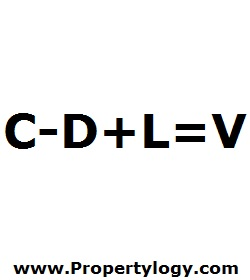Formula - Cost Approach Property Valuation | Propertylogy
Don't Miss

# Formula – Cost Approach Property Valuation

By on June 14, 2018C – D + L = V

C – Improvement costs

D – Depreciation

L – Land value

V – Property value

The cost approach is one of the most common methods of estimating the value of properties.

The answer is derived from asking the question “How much would it cost to build a similar property to the one in question?”.

Depreciation is a deduction in the equation as it represents the lesser condition of the property over time. And land value is added to the formula to properly factor in the property value as a whole consisting of improvements and land value.

Do be mindful that the actual cost of improvements will be subject to various variables that heavily depend on factors such as geography.

Send this to a friend